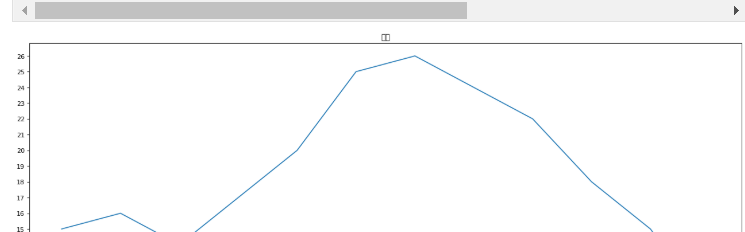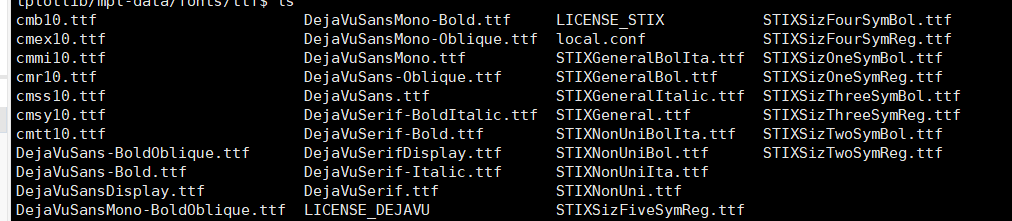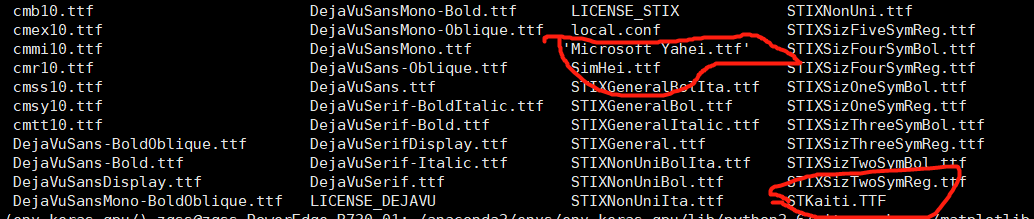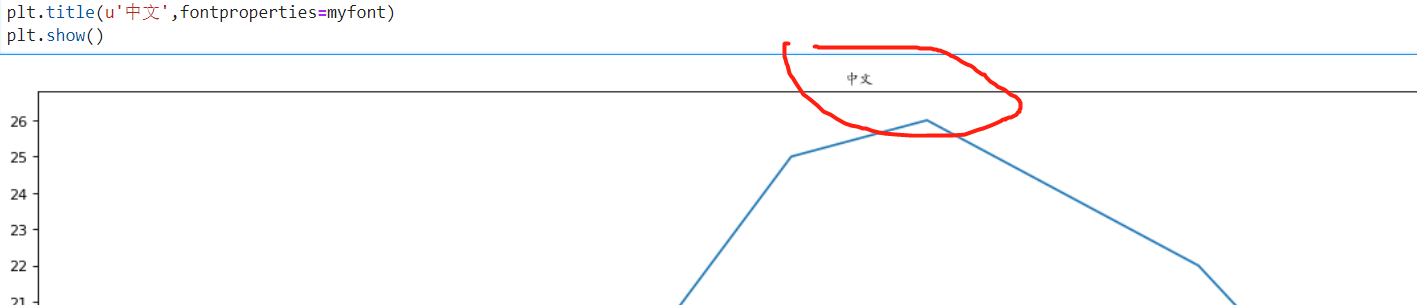# 解决在Linux系统下利用python matplotlib画图的中文显示为方框问题

• python3.6
• ubuntu
• conda3 下的matplotlib
• jupyterLab

• matplotlib画图，无法显示中文
• 不加字体时，代码以及图显示如下：
• import matplotlib.pyplot as plt
import random
from matplotlib.font_manager import FontProperties
from pylab import *

# 每隔两小时range(2， 26， 2) ,数据在x轴的数据， 可迭代
x = range(2, 26, 2)
y = [15, 16, 14, 17, 20, 25, 26, 24, 22, 18, 15, 10]

fig = plt.figure(figsize=(20, 8), dpi=80) # (20, 8)宽20，高8，dpi设置图片清晰度， 让图片更加清晰
plt.plot(x, y)

# 设置x轴, y轴的刻度
_xticks_labels = [i/2 for i in range(2, 49)]
plt.xticks(_xticks_labels[: :4]) # 设置步长
plt.yticks(range(min(y), max(y)+1))
plt.title(u'中文')
plt.show()

•• linux操作系统以及matplotlib的字体库中，没有可用的中文字体
• matplotlib包默认只支持ASCII码，不支持unicode码

1. 找到linux下matplotlib安装包下的字体文件库路径：

'/home/XXX/anaconda3/envs/env_keras_gpu/lib/python3.6/site-packages/matplotlib/mpl-data/fonts/ttf'2. 下载你需要的字体

3. 将你下载的字体放在文件夹下

'/home/XXX/anaconda3/envs/env_keras_gpu/lib/python3.6/site-packages/matplotlib/mpl-data/fonts/ttf'4. 字体下载好了，可以加入字体了

import matplotlib.pyplot as plt
import random
from matplotlib.font_manager import FontProperties

from pylab import *
fname = "/home/XXX/anaconda3/envs/env_keras_gpu/lib/python3.6/site-packages/matplotlib/mpl-data/fonts/ttf/STKaiti.TTF"
myfont = FontProperties(fname=fname)

# 每隔两小时range(2， 26， 2) ,数据在x轴的数据， 可迭代
x = range(2, 26, 2)
y = [15, 16, 14, 17, 20, 25, 26, 24, 22, 18, 15, 10]

fig = plt.figure(figsize=(20, 8), dpi=80) # (20, 8)宽20，高8，dpi设置图片清晰度， 让图片更加清晰
plt.plot(x, y)

# 设置x轴, y轴的刻度
_xticks_labels = [i/2 for i in range(2, 49)]
plt.xticks(_xticks_labels[: :4]) # 设置步长
plt.yticks(range(min(y), max(y)+1))
plt.title(u'中文',fontproperties=myfont)
plt.show()